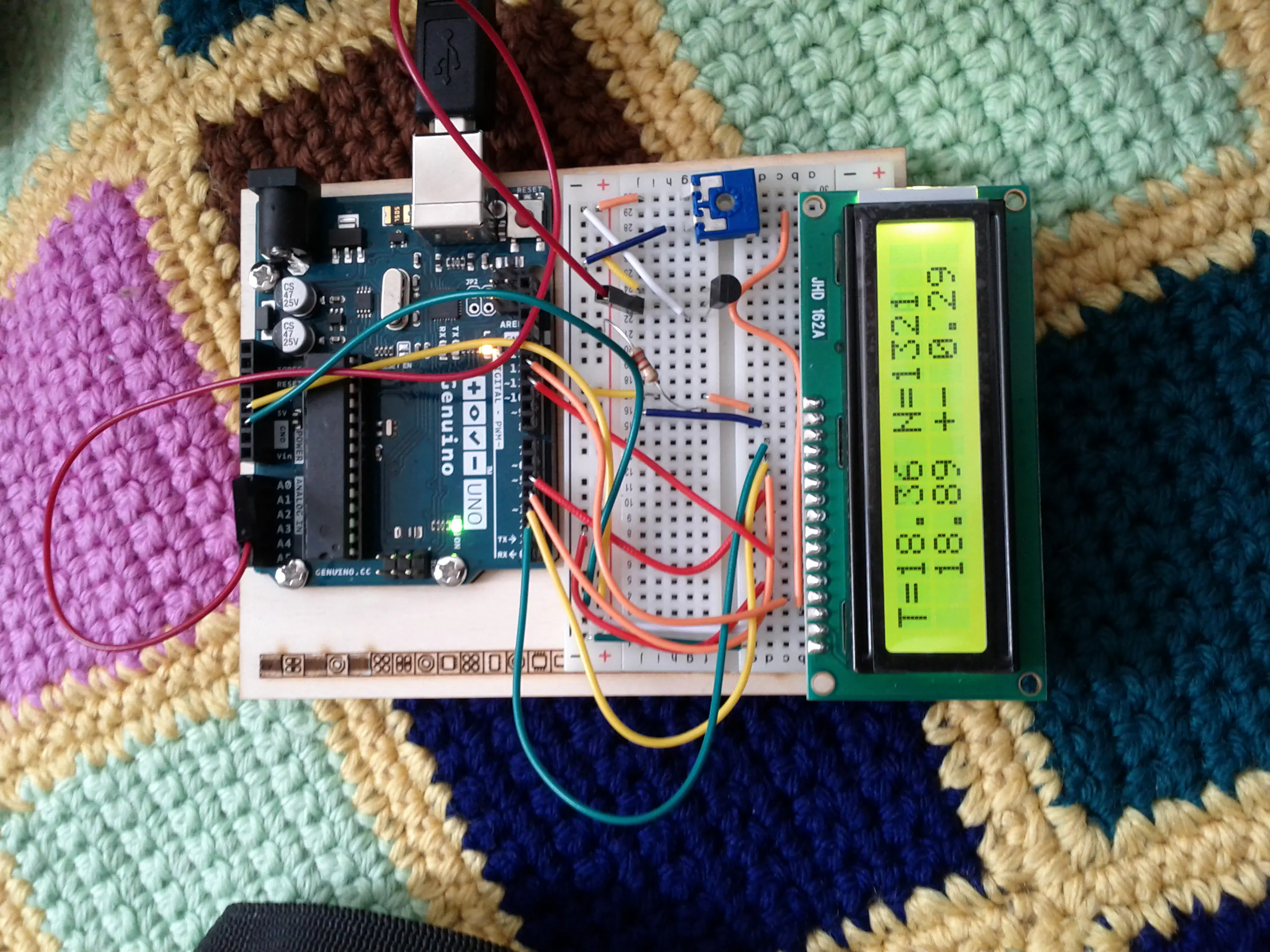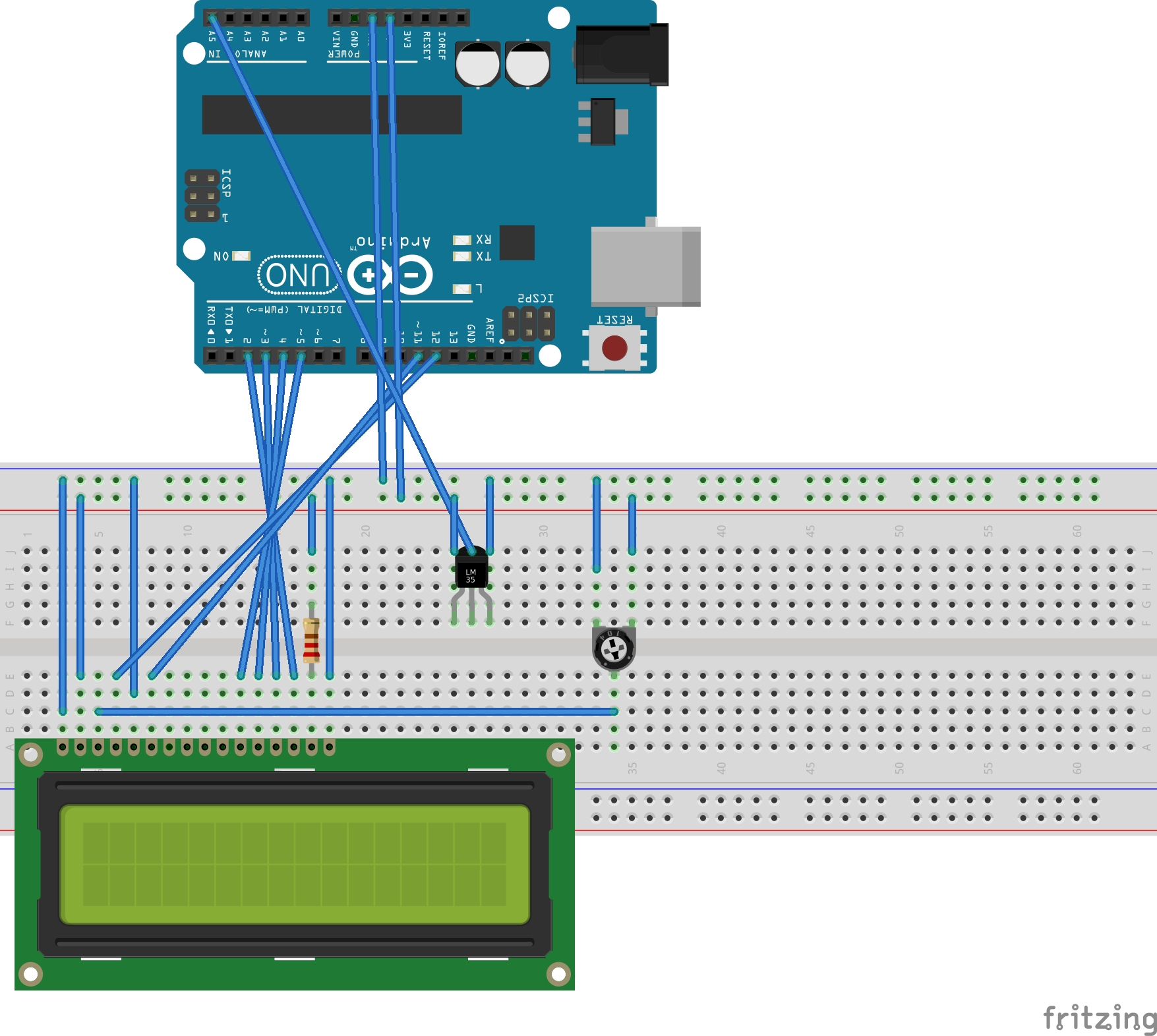# Simple Data Statistics (Temperature) © MIT

Arduino collects and calculates basic statistics on the acquired analog (temperature) data: average, standard deviation, min and max.

• 4,031 views
• 13 respects

## Components and supplies

When you use Arduino to sense with the digital inputs there are no doubts: it is either on or off. When it comes to analog input instead, the noise and/or other reasons make the reading oscillate. This project allows the calculation of a basic statistics summary on sampled data (average, standard deviation, min, max), without saving the whole bunch of data! So it is very useful on low resources hardware as Arduino. This can be applied to any analog quantity measured by an Arduino.

As an example I made a thermometer and connected Arduino to an LCD. This project does not include particular difficulties. To build it you may also refer to other projects/tutorials.

The software part is the main contribution of this project. Here how to use it:

``````#include "AvgStd.h"
AvgStd mySamples;
void setup(){
mySamples = AvgStd();
mySamples.setRejectionSigma(7.0);
}

void loop(){
float value = someArduinoSample(); // here you get a value of some
// kind from your arduino board.
// get the average and standard deviation, min, max and number of samplings;
float average = mySamples.getMean();
float std = mySamples.getStd();
float min = mySamples.getMin();
float max = mySamples.getMax();
int N = mySamples.getN();

}
``````

But here is how it all started...

Some time ago I was sick with some fever. The only thing I could do was to lay in bed and measure my body temperature. The thermometer took so little time to measure that I tried again and again. Each time the temperature was different.

I thought to measure a few times and then to calculate the average, to get a more stable result.

Doing it without a piece of paper starts to be a bit difficult when you have to deal with more than 5 samples (at least for me). I started thinking that there must be a way to update the last calculated average with the next sample.

I easily worked out the formula:

<T>_(N+1) = N/(N+1) * <T>_N + T_(N+1)/(N+1)

where <T>_(N+1) is the average calculated with N+1 samples and N is the number of samples. This formula says that we can calculate the next average by keeping in mind only 3 numbers: the previous average, the number of samplings and the last sample. That's nice!

I worked out also the rule for the standard deviation, or better for the variance.

var_(N+1) = var_N * ((N-1)/N) + (T_(N+1) - <T>_N)^2

Here, you need to remember 4 numbers, the previous average and variance, the last sample and the number of samplings.

This rules are very useful especially on low resource hardware because they do not require a lot of memory. Only 4 variables (3 floats and an int) have to be stored. I put them together in a simple project, because I think they can be useful for many.

I later found that this formulas were already known (guess what??) but it was nice to find them by myself!

## Code

##### AvgStd.inoC/C++
main file.
```#include "AvgStd.h"
#include <LiquidCrystal.h>

LiquidCrystal lcd(12,11,5,4,3,2);
const int sensorPin = A0;
AvgStd mySamples;

void setup(){
mySamples = AvgStd();
mySamples.setRejectionSigma(7.0);
Serial.begin(9600);
while (!Serial) {
; // wait for serial port to connect. Needed for native USB port only
}

// send an intro:
Serial.println("\n\nAvgStd:");
Serial.println();
lcd.begin(16, 2);
// Print a message to the LCD.
lcd.print("hello, world!");
}

void loop(){

// print string
//
lcdPrint(T);

//if reset button pressed
// mySamples.reset();
delay(1000);
}

// convert to V (ADC 1024 values, 5V max)
// float vr = ((float)v / 1024.0) * 5.0;
// T = ( v [V] - 0.5 V) * 100 [C]
return 100 * ((((float)v / 1024.0) * 5.0) - 0.5);
}

void serialPrint(float T){
Serial.print("T = " + String(T, 2));
Serial.println(" N = " + String(mySamples.getN()));
Serial.print("avg: " + String(mySamples.getMean(),2));
Serial.println(" +- " + String(mySamples.getStd(),2));
Serial.print("min: " + String(mySamples.getMin(),2));
Serial.println(" max: " + String(mySamples.getMax(),2));

}

void lcdPrint(float T){
lcd.clear();
// print first row with avg/std
lcd.setCursor(0,0);
String a = "T=" + String(mySamples.getMean(),2);
lcd.print(a);
lcd.setCursor(8,0);
a = "+- " + String(mySamples.getStd(),2);
lcd.print(a);
lcd.setCursor(15,0);
lcd.print( mySamples.getN()%2 == 0? "* " : "  " );
// second row alternates min/max with current reading
lcd.setCursor(0,1);
if ((mySamples.getN()/10)%2 == 0){
//display avg+std
lcd.setCursor(0,1);

a = "  " + String(T,2) + " N=" + String(mySamples.getN());
lcd.print(a);

} else {
// display min/max
a = "m " + String(mySamples.getMin(),2);
lcd.print(a);
lcd.setCursor(9,1);
a = "M " + String(mySamples.getMax(),2);
lcd.print(a);
}

}
```
##### AvgStd.hC/C++
Header for the simple iterative statistic package
```/**
* Library to calculate the average and standard deviation of a
* reading iteratively: without storing the whole data set.
*
* Stores:
* double average
* double variance
* double min
* double max
**/

/*

Permission is hereby granted, free of charge, to any person obtaining a copy of this software and associated documentation files (the "Software"), to deal in the Software without restriction, including without limitation the rights to use, copy, modify, merge, publish, distribute, sublicense, and/or sell copies of the Software, and to permit persons to whom the Software is furnished to do so, subject to the following conditions:

The above copyright notice and this permission notice shall be included in all copies or substantial portions of the Software.

THE SOFTWARE IS PROVIDED "AS IS", WITHOUT WARRANTY OF ANY KIND, EXPRESS OR IMPLIED, INCLUDING BUT NOT LIMITED TO THE WARRANTIES OF MERCHANTABILITY, FITNESS FOR A PARTICULAR PURPOSE AND NONINFRINGEMENT. IN NO EVENT SHALL THE AUTHORS OR COPYRIGHT HOLDERS BE LIABLE FOR ANY CLAIM, DAMAGES OR OTHER LIABILITY, WHETHER IN AN ACTION OF CONTRACT, TORT OR OTHERWISE, ARISING FROM, OUT OF OR IN CONNECTION WITH THE SOFTWARE OR THE USE OR OTHER DEALINGS IN THE SOFTWARE.
*/

#ifndef AVGSTD_H
#define AVGSTD_H

#include "Arduino.h"

class AvgStd {
public:
float getMean();
float getStd();
float getMin();
float getMax();
float getVariance();
unsigned int getN();
void reset();
void setRejectionSigma(float);
int getTrend();
void setTrendInterval(int);
void setSamplingInterval(int);
AvgStd();
private:
float min, max, var, avg, r_sigma;
unsigned int N;

};

#endif
```
##### AvgStd.cppC/C++
implementation of simple statistics
```/**
* Library to calculate the average and standard deviation of a
* reading iteratively: without storing the whole data set.
*
* Stores:
* double average
* double variance
* double min
* double max
**/

/*

Permission is hereby granted, free of charge, to any person obtaining a copy of this software and associated documentation files (the "Software"), to deal in the Software without restriction, including without limitation the rights to use, copy, modify, merge, publish, distribute, sublicense, and/or sell copies of the Software, and to permit persons to whom the Software is furnished to do so, subject to the following conditions:

The above copyright notice and this permission notice shall be included in all copies or substantial portions of the Software.

THE SOFTWARE IS PROVIDED "AS IS", WITHOUT WARRANTY OF ANY KIND, EXPRESS OR IMPLIED, INCLUDING BUT NOT LIMITED TO THE WARRANTIES OF MERCHANTABILITY, FITNESS FOR A PARTICULAR PURPOSE AND NONINFRINGEMENT. IN NO EVENT SHALL THE AUTHORS OR COPYRIGHT HOLDERS BE LIABLE FOR ANY CLAIM, DAMAGES OR OTHER LIABILITY, WHETHER IN AN ACTION OF CONTRACT, TORT OR OTHERWISE, ARISING FROM, OUT OF OR IN CONNECTION WITH THE SOFTWARE OR THE USE OR OTHER DEALINGS IN THE SOFTWARE.
*/

#include "AvgStd.h"
#include <math.h>

AvgStd::AvgStd(){
AvgStd::reset();
}

if (N < 10)
else {
if (r_sigma == -1)
else {
if (abs(avg - val) <= (r_sigma * sqrt(var)) )
}
}

}

if (N == 0) {
avg = val;
min = val;
max = val;
} else if (N == 1) {
//set min/max
max = val > max? val : max;
min = val < min? val : min;

float thisavg = (avg + val)/2;
// initial value is in avg
var = (avg - thisavg)*(avg-thisavg) + (val - thisavg) * (val-thisavg);
avg = thisavg;
} else {
// set min/max
max = val > max? val : max;
min = val < min? val : min;

float M = (float)N;

var = var * ((M-1)/M) + ((val - avg)*(val - avg)/(M+1)) ;

avg = avg * (M/(M+1)) + val / (M+1);
}
N++;
}

void AvgStd::reset(){
N = 0;
avg = 0;
var = 0;
min = 0;
max = 0;
r_sigma = -1;
}

float AvgStd::getMean(){return avg;}
float AvgStd::getStd() {
float ret = -1;
if (N>1)
ret = sqrt(var);
return ret;
}
float AvgStd::getVariance() {return var;}
unsigned int AvgStd::getN(){return N;}
float AvgStd::getMin() {return min;}
float AvgStd::getMax() {return max;}

void AvgStd::setRejectionSigma(float sigmas){
r_sigma = sigmas;
};

```

## Schematics

There is a Genuino board, a LCD 16x2 and a LMP36 temperature sensors and a lot of wires!#### Temperature and Humidity Data Logger

Project tutorial by Wimpie van den Berg

• 32,438 views
• 31 respects

#### Temperature + Humidity on LCD

Project showcase by interpeo

• 28,965 views
• 61 respects

#### Simple Ultrasonic Distance Measurer With LCD Display

Project showcase by onatto22

• 13,231 views
• 34 respects

#### Portable Temperature Station

Project tutorial by Isaac100

• 11,481 views
• 40 respects

#### Temperature & Humidity Data Logging

Project tutorial by MatrixOrbital

• 4,059 views